## Friday, September 4, 2015

### Plotting stochastic-map tree with an outline; splitting vertical lines in a plotted tree by the mapped states of the daughters

Today the following comment was posted on the phytools blog comments page:

“Thanks for providing such great resources. I have a couple of questions that I cannot seem to find answers for (and hope I have not missed anything obvious).

Is it possible to outline branches in plotSimmap? I would like to use black, grey and white for simplicity, but only if I can see the white branches…

Also, I notice that the colour at the nodes (vertical branches on a square phylogeny) are the colour of just one of the tipwards branches (the bottom branch). Is it possible for the nodes to be coloured equally by the two branches?”

First question (Is it possible to outline branches in plotSimmap? I would like to use black, grey and white for simplicity, but only if I can see the white branches…). This is not too difficult, in fact. Here is a quick demo:

``````set.seed(13)
library(phytools)
## simulate some data:
Q<-matrix(c(-2,1,1,1,-2,1,1,1,-2)/2,3,3,
dimnames=list(letters[1:3],letters[1:3]))
tree<-sim.history(pbtree(n=26,tip.label=LETTERS,scale=1),Q,anc="a")
``````
``````## Done simulation(s).
``````
``````plot(tree)
``````
``````## no colors provided. using the following legend:
##        a        b        c
##  "black"    "red" "green3"
``````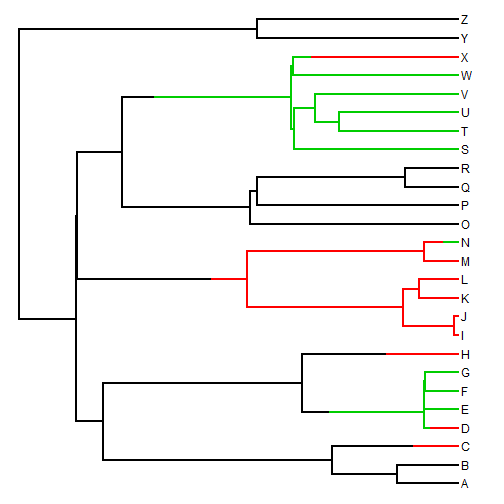``````## now let's do it with an outline:
par(fg="transparent")
plotTree(tree,lwd=5,ylim=c(-1,Ntip(tree)))
par(fg="black")
colors=setNames(c("black","grey","white"),
sort(unique(getStates(tree,"tips"))))
colors
``````
``````##       a       b       c
## "black"  "grey" "white"
``````
``````plot(tree,lwd=3,colors=colors,add=TRUE,ylim=c(-1,Ntip(tree)))
prompt=FALSE,vertical=FALSE)
``````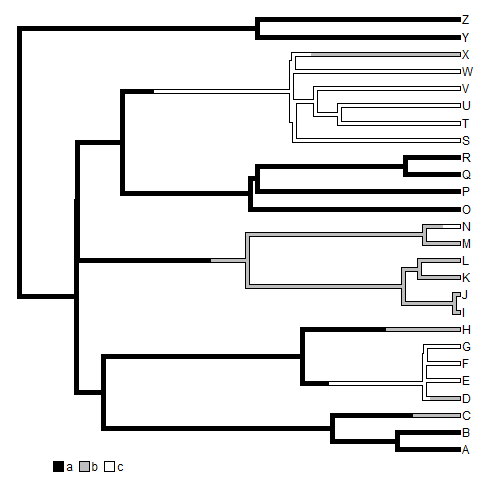The second question was as follows: Also, I notice that the colour at the nodes (vertical branches on a square phylogeny) are the colour of just one of the tipwards branches (the bottom branch). Is it possible for the nodes to be coloured equally by the two branches?

Well, if the plotted tree is a stochastic map tree, then it is theoretically impossible that the character changes state precisely at a node. However, `plotSimmap` can be used to plot trees that are not stochastic map trees, including trees in which the edge states have been set using `paintBranches` and `paintSubTree`.

``````tree<-pbtree(n=26,tip.label=LETTERS,scale=1)
plotTree(tree,node.numbers=TRUE)
``````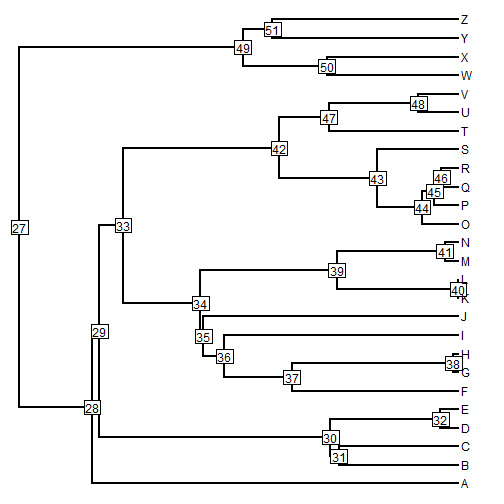``````tree<-paintSubTree(tree,33,state="b",anc.state="a")
tree<-paintSubTree(tree,42,state="c",stem=TRUE)
colors<-setNames(c("black","blue","red"),letters[1:3])
plot(tree,colors,lwd=3)
``````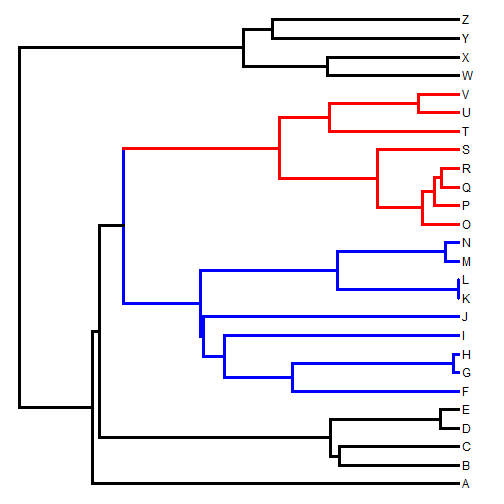So here we see that the vertical lines connecting edges have one or the other state, rather than spltting between the two daughter edge.

The following is a custom function to split the edge color between the two daughters when (and only when) the state changes exactly at a node:

``````splitEdgeColor<-function(tree,colors,lwd=2){
obj<-get("last_plot.phylo",envir=.PlotPhyloEnv)
for(i in 1:tree\$Nnode+Ntip(tree)){
daughters<-tree\$edge[which(tree\$edge[,1]==i),2]
for(j in 1:length(daughters)){
jj<-which(tree\$edge[,2]==daughters[j])
color<-if(tree\$maps[[jj]]==0) colors[names(tree\$maps[[jj]])] else colors[names(tree\$maps[[jj]])]
lines(rep(obj\$xx[i],2),obj\$yy[c(i,daughters[j])],col=color,lwd=lwd)
}
}
}
``````

Let's try it:

``````plot(tree,colors,lwd=3)
splitEdgeColor(tree,colors,lwd=3)
``````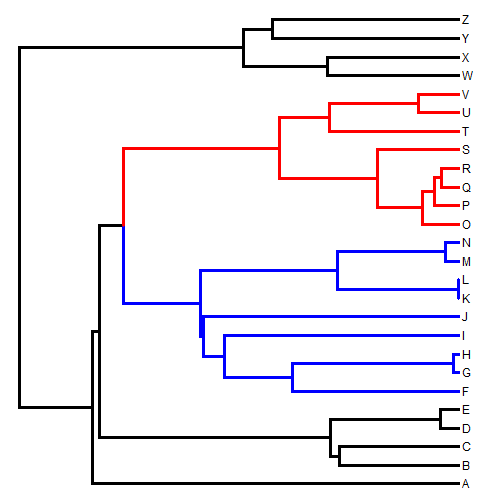We can also try it with `paintBranches`:

``````tree<-pbtree(n=26,tip.label=LETTERS,scale=1)
b<-sample(tree\$edge[,2],10)
c<-sample(setdiff(tree\$edge[,2],b),10)
tree<-paintBranches(tree,b,"b","a")
tree<-paintBranches(tree,c,"c")
plot(tree,colors,lwd=3)
splitEdgeColor(tree,colors,lwd=3)
``````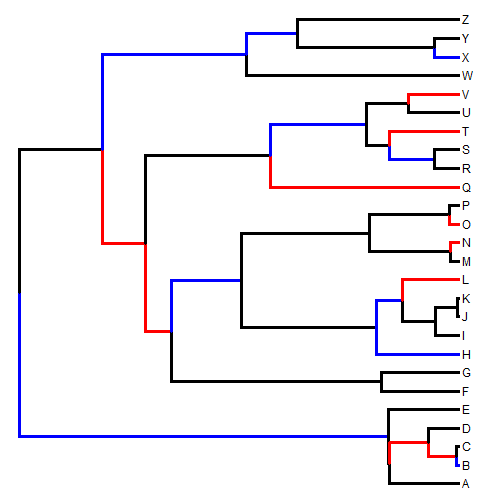Nice. It seems to work.